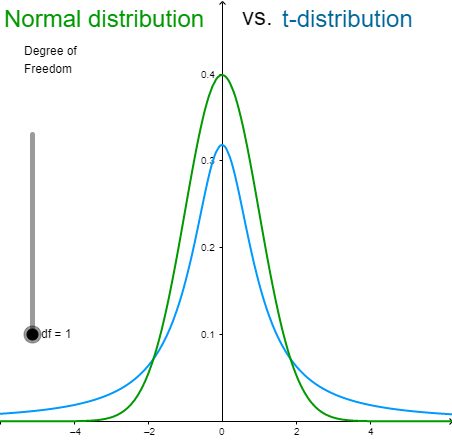## 24.1 Degrees of Freedom (df)

As the number of degrees of freedom (df) grows, the $$t$$ distribution approaches the standard normal distribution ($$N(0,1)$$), and in fact the approximation is quite close for $$\text{df} \geq 30$$.4

Figure below shows a comparison of $$t$$ distributions with 1, 5, 10, and 30 df and the standard normal distribution. The distribution with the lowest peak is the 1 df distribution, the next lowest is 5 df, the lowest after that is 10 df, and the highest is the standard normal distribution.

Recall that with few degrees of freedom, the values of $$t$$ are much higher than the corresponding values for a normal distribution and that the difference decreases as the degrees of freedom increase.5

Tip
You can use this app Normal Distribution vs. t-distribution to know more about Normal Distribution vs. t-distribution.